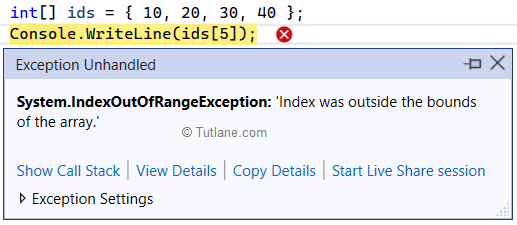# IndexOutOfRangeException in C# with Examples

By : Suresh Dasari
Posted On : 27-May-2023

Here we will learn what is indexoutofrangeexception in c#, how to handle or avoid indexoutofrangeexception in c#, and how to fix indexoutofrangeexception in c# with examples.

## What is IndexOutOfRangeException in C#?

In c#, IndexOutOfRangeException is an exception that will occur when we try to access the elements of an array or collection with an index that is outside of the valid range.

In application, when we get an IndexOutOfRangeExpcetion it indicates that we are trying to access the collection object elements using the invalid index that could result a runtime error (System.IndexOutOfRangeException: 'Index was outside the bounds of the array.') as shown below.## Possible Ways to Get IndexOutOfRangeException in C#

Following are some possible ways of getting the IndexOutOfRangeException error in c#.

Example1: When you try to access the array elements using an index value more than its total elements.

using System;

namespace Tutlane
{
class Program
{
static void Main(string[] args)
{
int[] ids = { 10, 20, 30, 40 };
Console.WriteLine(ids); // Throws IndexOutOfRangeException
}
}
}

In this example, the ids array contains a total of 4 elements which means we can access the array elements using a valid range of 0 to 3. Here, we are trying to access the 6th element of an array using ids which is outside of the valid range due to that we will get System.IndexOutOfRangeException: 'Index was outside the bounds of the array.'.

Example2: When you try to access the list elements using an index value more than its valid range.

using System;
using System.Collections.Generic;

namespace Tutlane
{
class Program
{
static void Main(string[] args)
{
List lst = new List { 100, 200, 300};
Console.WriteLine(lst); // Throws IndexOutOfRangeException
}
}
}

In this example, the lst list contains a total of 3 elements which means we can access the list elements using the valid range 0 to 2. Here, we are trying to access the 4th element of an array using lst which is outside of the valid range due to that we will get System.ArgumentOutOfRangeException: 'Index was out of range. Must be non-negative and less than the size of the collection. (Parameter 'index')'.

Example3: When you try to loop through the array or collection elements using index value more than its total elements.

using System;

namespace Tutlane
{
class Program
{
static void Main(string[] args)
{
int[] ids = { 10, 20, 30, 40 };
for(int i = 0; i <= ids.Length; i++)
{
Console.WriteLine(ids[i]); // Throws IndexOutOfRangeException
}
}
}
}

In this example, we are looping through the ids array using array length (ids.Length) with condition <= instead of < which is one element beyond the valid range due to that we will get System.IndexOutOfRangeException: 'Index was outside the bounds of the array.'.

## How to Handle IndexOutOfRangeException in C#

In c#, we have many ways to handle or prevent the “IndexOutOfRangeException” error.

Solution1: To access elements from collection objects, the index will start from 0 so while accessing the elements we need to make sure that the upper bound of the collection is one less than the total number of elements.

using System;

namespace Tutlane
{
class Program
{
static void Main(string[] args)
{
int[] ids = { 10, 20, 30, 40 };
for (int i = 0; i < ids.Length; i++)
{
Console.WriteLine(ids[i]);
}
}
}
}

In this example, we are looping through the ids array using array length (ids.Length) with condition < which is one element less than the total elements. This will prevent IndexOutOfRange Exception from the application.

Solution2: If you use for loop in c#, you need to consider using the index of the array or collection to loop through the elements. Instead, use foreach loop to avoid the index dependency and directly access the elements.

using System;

namespace Tutlane
{
class Program
{
static void Main(string[] args)
{
int[] ids = { 10, 20, 30, 40 };
foreach (var id in ids)
{
Console.WriteLine(id);
}